## Excel ASC Function

The ASC function returns the ASCII code for the first character of a string. It is mostly used as a VBA function in Excel.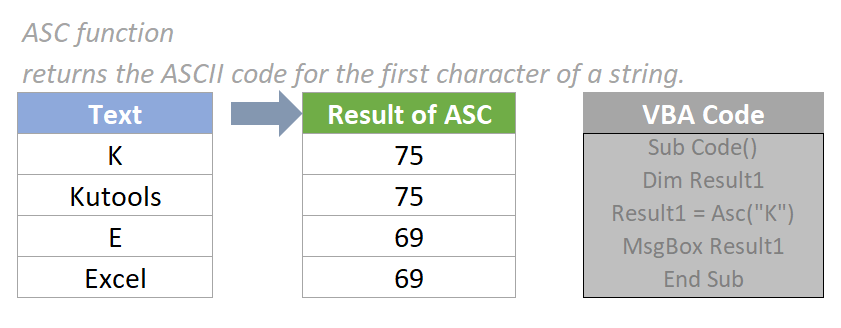=ASC(text)

#### Arguments

• Text (required):The text or a reference to a cell that contains the text you want to convert to ASCII code..

#### Return Value

The ASC function returns a numeric value.

#### Function notes

1. The text string supplied to ASC function in VBA can be any valid string, but it only calculates the first character of the string.
2. ASC function is case sensitive.
3. The ASCII code returned by ASC function is the integer part of a Decimal number, range from 0 to 255. Just like screenshot below shows. For more details about ASCII code, please refer to this article: https://www.ascii-code.com/.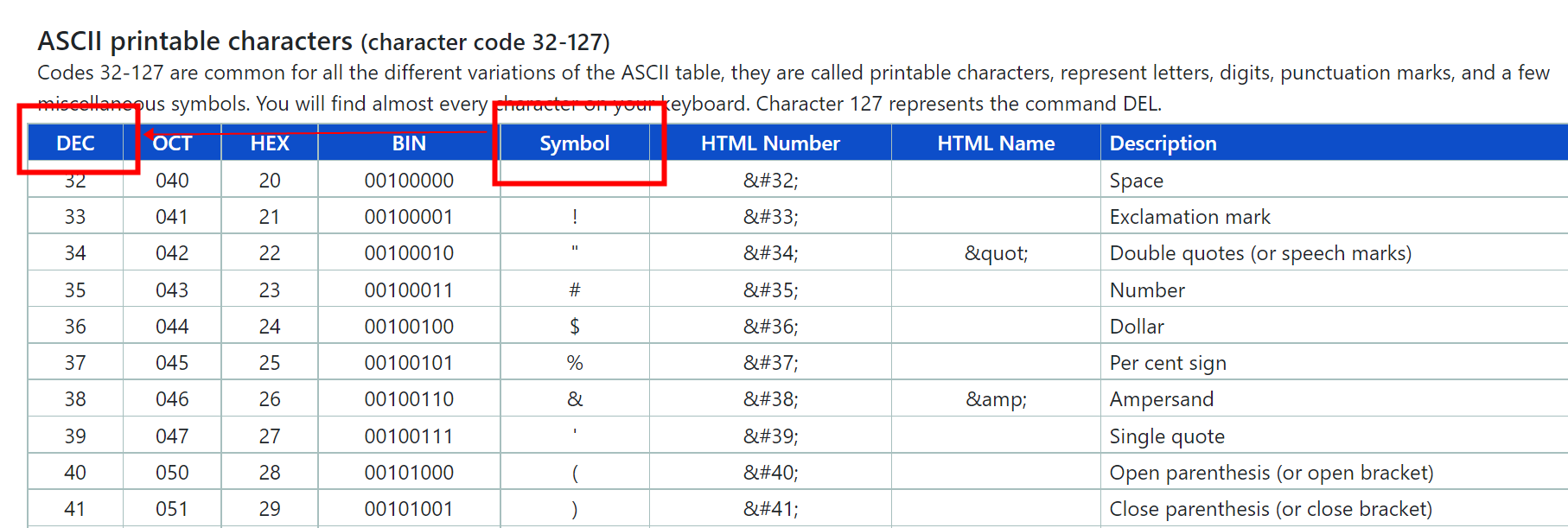4. The ASCII codes for A-Z returned by ASC function in VBA are 65-90, and for a-z are 97-122.

#### Example

In this case, there are some test strings in the table below. To calculate the ASCII code of those text strings, please do as follows:

1. Press the Alt + F11 keys to open the Microsoft Visual Basic for Applications window.

2. In the Microsoft Visual Basic for Applications window, please click Insert > Module to open the Module window. Then copy and paste the below VBA code into the window.

VBA: calculate the ASCII code

``````Sub Code()
Dim Result1
Result1 = Asc("K")
MsgBox Result1
End Sub``````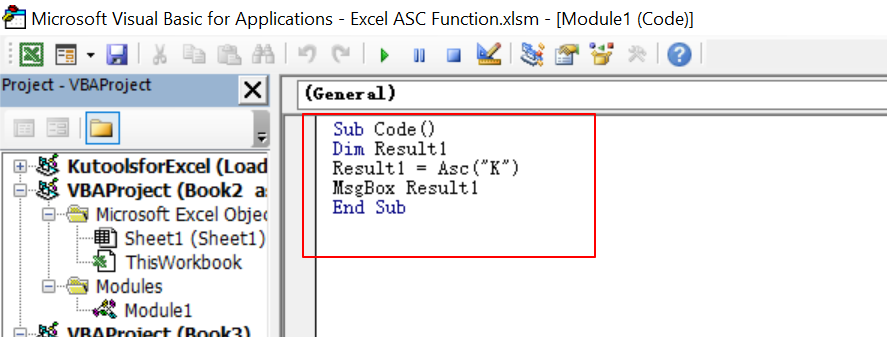3. Press the F5 key to run the code. And a Microsoft Excel dialog box pops up, displaying the ASSII code result of text “K”, which is 75.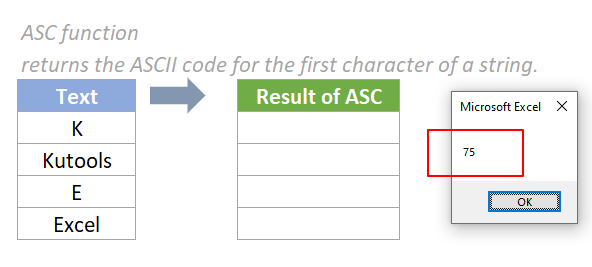4. Input the result “75” into cell D5 so the result can be recorded.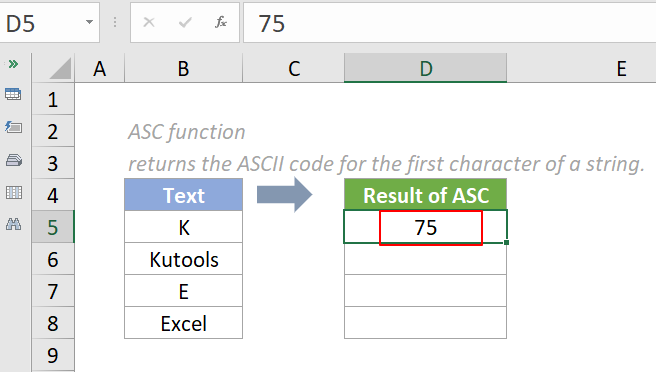5. To get the rest of the results. We need to change the text in the ASC function in VBA accordingly. For example, to know the ASSII code of text “Kutools”, the VBA is like this:

VBA: calculate the ASCII code

``````Sub Code()
Dim Result2
Result2 = Asc("Kutools")
MsgBox Result2
End Sub
``````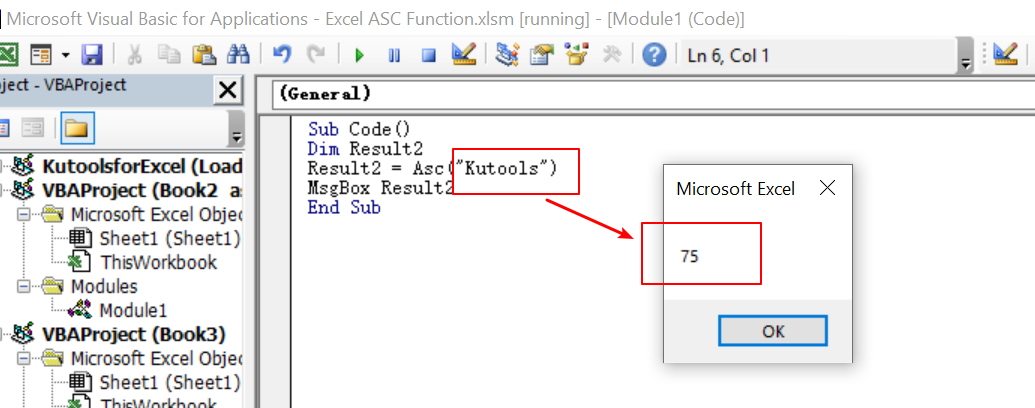You will notice that the result of text “Kutools” is the same as the one of text “K”.

6. Repeat the steps above to get the rest of the results.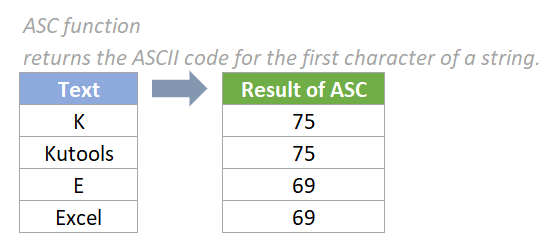Notes:

1. If the supplied string is blank, it returns a run-time error.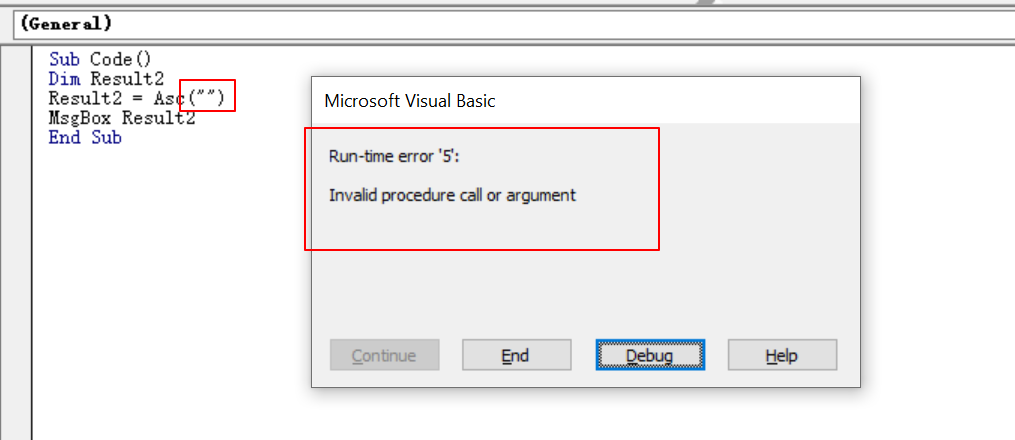2. If the supplied text is an empty space, it returns 32.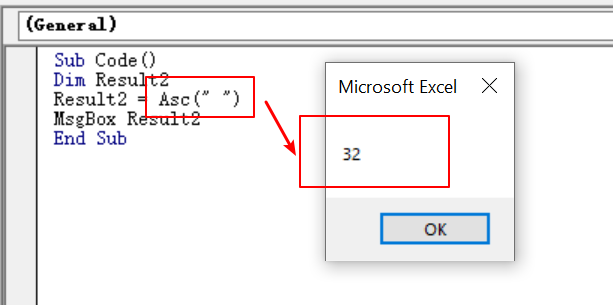3. As the ASC function is case sensitive, it returns different results for the same character in different cases, such as the characters E and e, ASC returns the results as 69 and 101 separately.

#### Relative Functions:

• Excel EVEN Function
The EVEN function rounds numbers away from zero to the nearest even integer.

• Excel EXP Function
The EXP function returns the result of the constant e raised to the nth power.